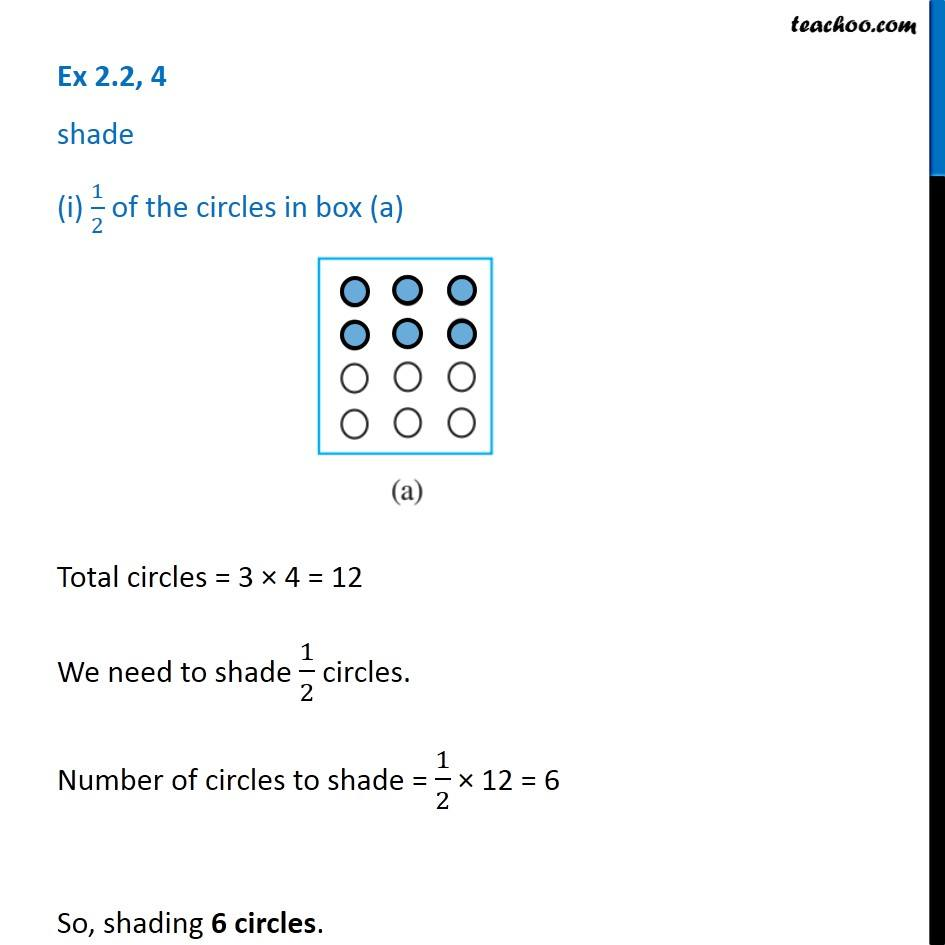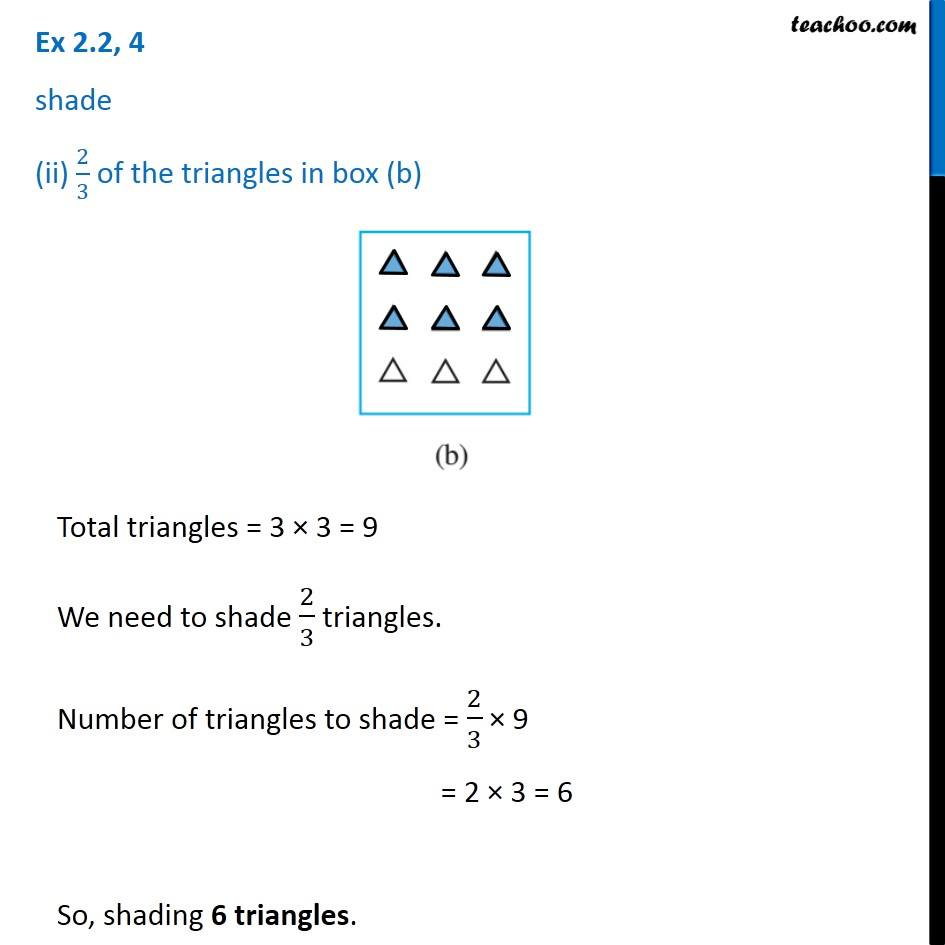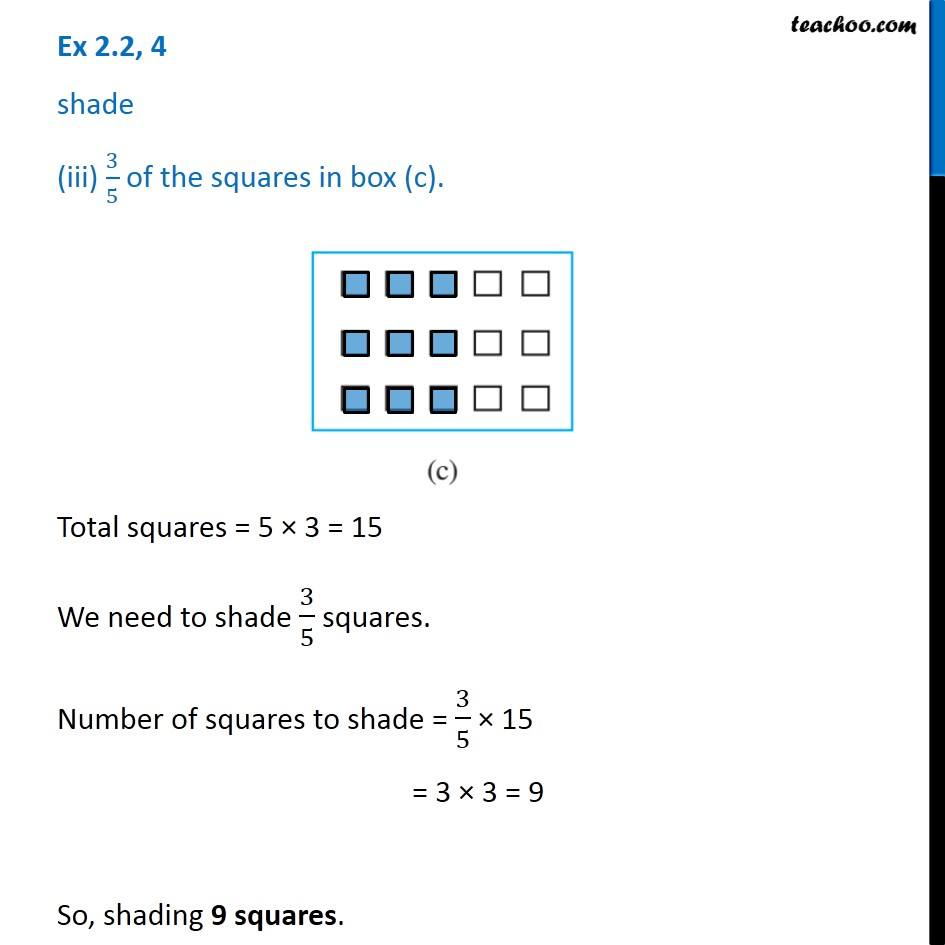1. Chapter 2 Class 7 Fractions and Decimals
2. Serial order wise
3. Ex 2.2

Transcript

Ex 2.2, 4 shade (i) 1/2 of the circles in box (a) Total circles = 3 × 4 = 12 We need to shade 1/2 circles. Number of circles to shade = 1/2 × 12 = 6 So, shading 6 circles. Ex 2.2, 4 shade (ii) 2/3 of the triangles in box (b)Total triangles = 3 × 3 = 9 We need to shade 2/3 triangles. Number of triangles to shade = 2/3 × 9 = 2 × 3 = 6 So, shading 6 triangles. Ex 2.2, 4 shade (iii) 3/5 of the squares in box (c). Total squares = 5 × 3 = 15 We need to shade 3/5 squares. Number of squares to shade = 3/5 × 15 = 3 × 3 = 9 So, shading 9 squares.

Ex 2.2